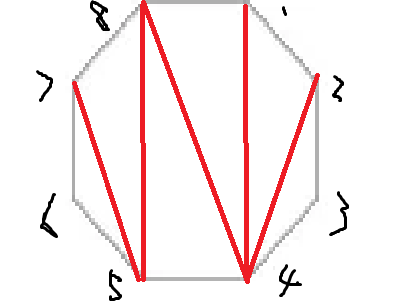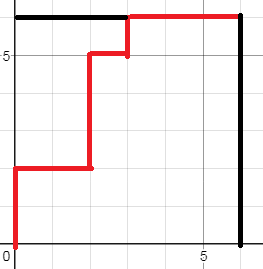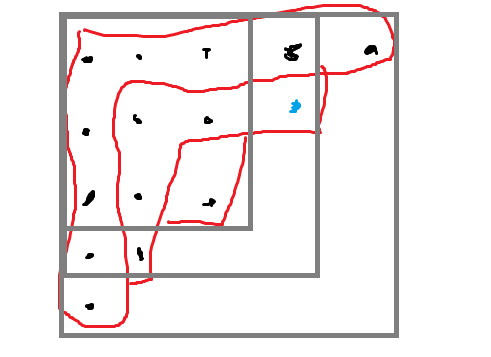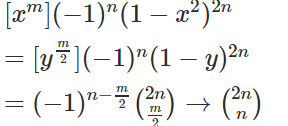is a collection of combinatorial objects.

=> and are combinatorically isomorphic

if there exist a size-preserving bijection between and

#### Q1

Prove that number of Dyck Paths equals to number of triangulations of a n-polygon1. Label all the points

2. if is an edge, , then we draw an edge

3. Finish by drawing an

#### Q2

Find the value of

take

#### HW

Find the value of

#### Q5

Provem is an even number#### Q6

Prove (Euler's Identity)

Prove

#### Thm

The OGF's for partitions

using parts in ;

partitions with largest part ;

all partitions,are

#### Def

a run is a maximal increasing string in the word

84135726 has 4 runs: 8, 4, 1357, 26

The Eulerian number

is the number of permutations of containing runs.

#### Thm (Worpitzky's Identity)

: number of functions from to

boxes, there can be nothing in a box, or some balls in a box. If there are some balls in a box, then list the balls from small to large, like:

separates, runs, so add separates, there are ways